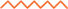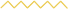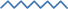Select Page

# Get a QuoteSof’Fall, Inc. has excellent representatives ready to serve you. Complete the quote request, and one of our representatives will help you in planning, making recreational surfacing recommendations, providing installation guidance and much more!

Use the material calculation chart below to figure the cubic yards OR send us the depth and square feet and we will contact you with an estimate.

Get a Quote

How many cubic yards do you need?

DON'T KNOW THE CUBIC YARDS? TELL US THE DEPTH AND THE SQUARE FOOTAGE

## Sof'Fall Material Calculation

The amount of Sof'Fall Engineered Wood Fiber necessary to provide the approximate depth after compaction is as follows:

#### DEPTH8"

9"

10"

11"

12"

#### PLAYGROUND AREAPer 1,000 sq ft

Per 1,000 sq ft

Per 1,000 sq ft

Per 1,000 sq ft

Per 1,000 sq ft

#### QUANTITY33 cubic yards

37 cubic yards

41 cubic yards

45 cubic yards

50 cubic yards

## Sof’Bounce Material Calculation

#### CRITICAL FALL HEIGHTS

INCHES COMPACTED CRITICAL FALL HEIGHT
4" = 6'
6" = 13'
12" = 25'
*Sof'Bounce minimum depth is 4 inches.

#### depth conversion

INCHES CONVERSION RATE
1" = 0.083'
2" = 0.166'
3" = 0.25'
4" = 0.33'
5" = 0.41'
6" = 0.5'
7" = 0.583'
8" = 0.666'
9" = 0.75'
10" = 0.83'
11" = 0.916'
12" = 1'

## Sof’Bounce Conversion Chart

STEP 1Playground Area

(LENGTH) X (WIDTH) = (SQUARE FOOTAGE)

STEP 2Cubic Feet

(SQUARE FOOTAGE) X (DEPTH) = (CUBIC FEET)

STEP 3Number of Super Sacks Needed

(CUBIC FEET) / 80 = (NUMBER OF SUPER SACKS)

STEP 4CALCULATION EXAMPLE

Your playground dimensions are 30 ft. long by 30 ft. wide which equates to 900 total square feet. Your critical fall height is 12 feet. Identify material depth for 12 ft. fall height on the critical fall height chart and add 2 inches for compaction, equaling 8″ of material required. To calculate cubic feet, multiply 900 sq. ft. by the depth conversion for 8″ of material or .666. Depending on how you would like your Sof’Bounce delivered, divide the cubic feet by the super sack formula. In this example, the playground area requires 8 super sacks (2000 lb).

Product Specifications

Sof’Bounce Material Calculation

# We've been catching children when they fall for over 27 Years

Get in touch with us to learn why our play area surface products are your best option.